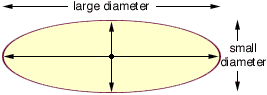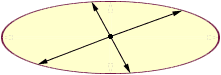Is there a such thing as a diameter of a oval? If not, is there a way to get the circumference? Tim K-5 Student Hi Tim, An ellipse is a stretched out circle. When you study algebra you will learn that its equation is  x2/a2 + y2/b2 = 1, for some positive numbers a, b. The graph of an ellipse looks like an oval as in the diagram below.I have always considered an ellipse to have two diameters as in the diagram but my dictionary says that a diameter is any chord passing through the center. Thus, according to my dictionary, the chords in the diagram below are also diameters.To find its circumference is much more difficult. You need at least two semesters of calculus to understand how to calculate it. So, in short, there is a formula that calculates the circumference of an ellipse, but it is pages long (just the formula). In practice if you need to find the circumference of an ellipse the usual method is to approximate it. Cheers, Claude and Penny Go to Math Central